This resource function is obsolete. Use the built-in symbol SimpleGraphQ instead.

Function Repository Resource:

# SimplicalGraphQ

Test whether a graph is simplical

Contributed by: Jon McLoone
 ResourceFunction["SimplicalGraphQ"][gr] tests whether the graph gr is simplical.

## Details

A graph is simplical if no edge starts and ends at the same vertex, and no two edges have the same pair of vertices.

## Examples

### Basic Examples (3)

If more than one edge joins the same pair of vertices then the graph is not simplical:

 In:=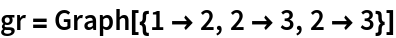Out=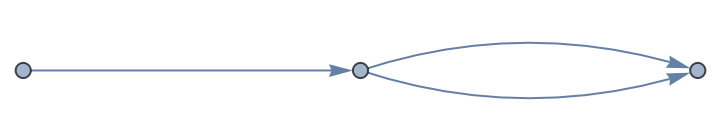In:=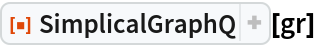Out=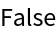Self-connected vertices are not simplical:

 In:=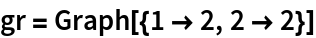Out=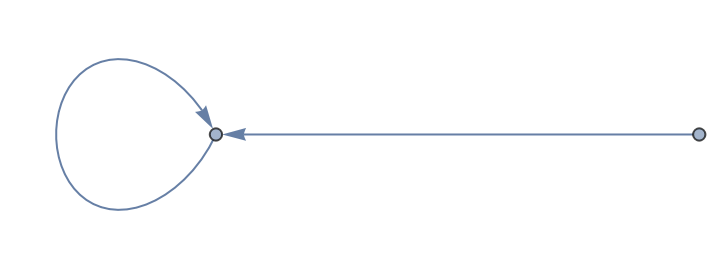In:=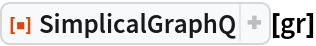Out=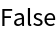The Petersen graph is simplicial:

 In:=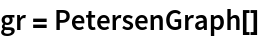Out=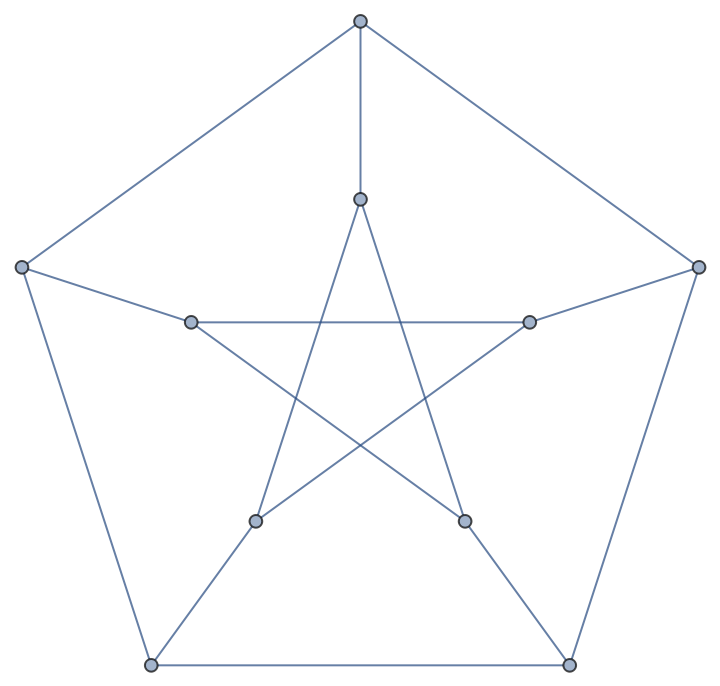In:=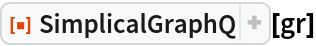Out=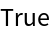Jon McLoone

## Version History

• 1.0.0 – 25 March 2022